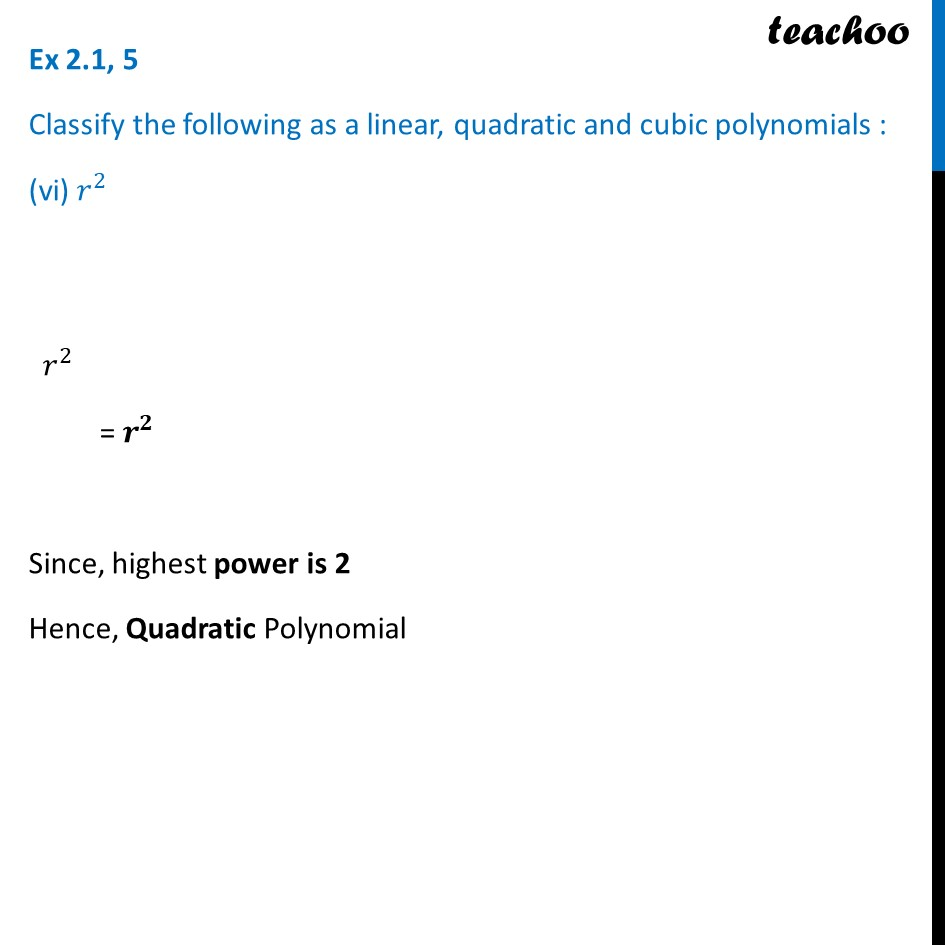1. Chapter 2 Class 9 Polynomials (Term 2)
2. Serial order wise
3. Ex 2.1

Transcript

Ex 2.1, 5 Classify the following as a linear, quadratic and cubic polynomials : (vi) 𝑟^2 𝑟^2 = 𝒓^𝟐 Since, highest power is 2 Hence, Quadratic Polynomial

Ex 2.1

Chapter 2 Class 9 Polynomials (Term 2)
Serial order wise